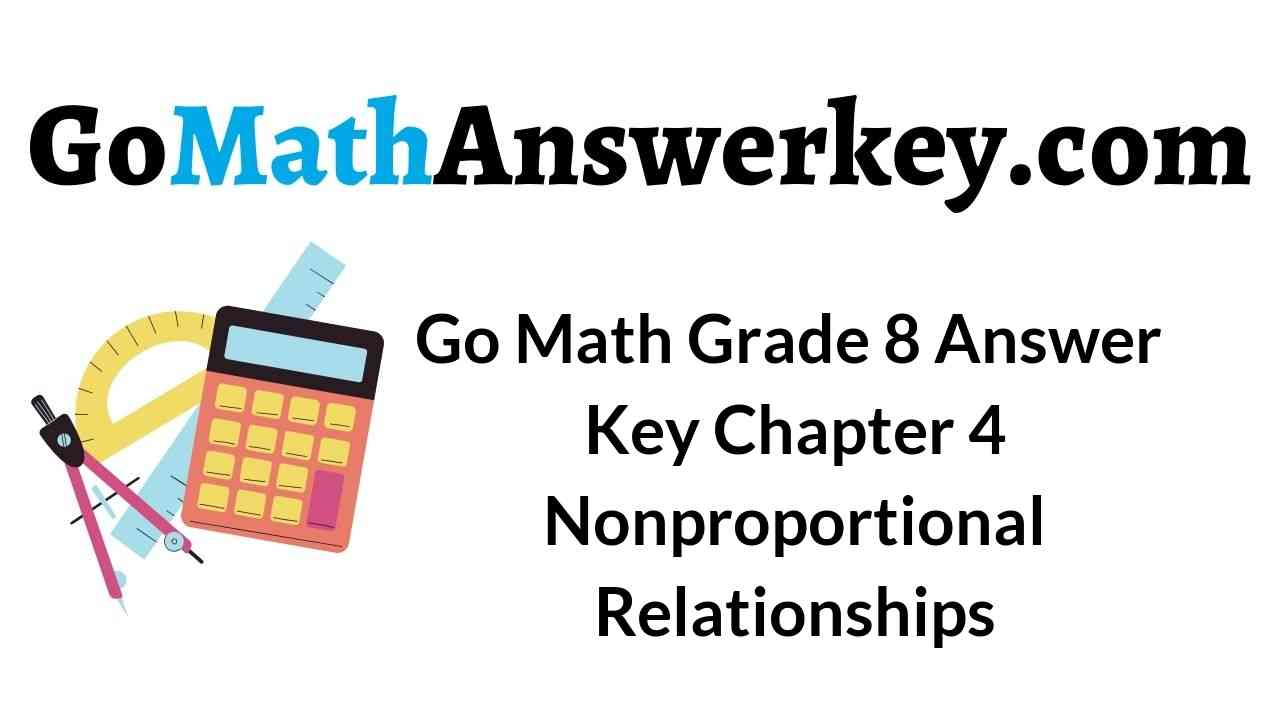The best Go Math Grade 8 Answer Key Chapter 4 Nonproportional Relationships PDF for more people who are seeking for math learning in an easy way. Find the top-suggested ways of math problem-solving methods and learn the best way to solve math. The list of all practice questions of Go Math Grade 8 Answer Key are given here in this article. The students can find and practice all the questions to score the good marks in the exam.

You can enjoy solving math problems with the help of Go Math Grade 8 Answer Key Chapter 4 Nonproportional Relationships. Download Go Math Grade 8 Chapter 4 Nonproportional Relationships Solution Key. Many students refer to HMH Go Math Grade 8 Chapter 4 Answer Key for the best practice.

Lesson 1: Representing Linear Nonproportional Relationships

Lesson 2: Determining Slope and y-intercept

Lesson 3: Graphing Linear Nonproportional Relationships Using Slope and y-intercept

Lesson 4: Proportional and Nonproportional Situations

Lesson 5: Representing Linear Nonproportional Relationships – Model Quiz

Mixed Review

### Guided Practice – Representing Linear Nonproportional Relationships – Page No. 98

Make a table of values for each equation.

Question 1.
y = 2x + 5Type below:
____________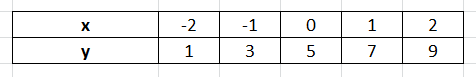Explanation:
y = 2x + 5
Choose several values for x and substitute in the equation to find y.
x = 2(-2) + 5 = 1
x = 2(-1) + 5 = 3
x = 2(0) + 5 = 5
x = 2(1) + 5 = 7
x = 2(2) + 5 = 9

Graphing Linear Nonproportional Relationships Worksheet Answers Question 2.
y = $$\frac{3}{8}$$x − 5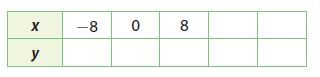Type below:
____________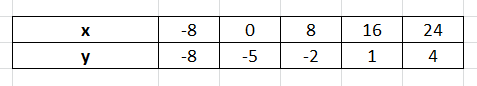Explanation:
y = $$\frac{3}{8}$$x − 5
Choose several values for x and substitute in the equation to find y.
x = 3/8(-8) – 5 = -8
x = 3/8(0) – 5 = -5
x = 3/8(8) – 5 = -2
x = 3/8(16) – 5 = 1
x = 3/8(24) – 5 = 4

Explain why each relationship is not proportional.

Question 3.First calculate $$\frac{y}{x}$$ for the values in the table.
____________

The relationship is not proportional

Explanation:
Find y/x
3/0 = undefined
7/2 = 3.5
11/4 = 2.75
15/6 = 2.5
19/8 = 2.375
The ratio is not constant, hence relationship is not proportional.

Question 4.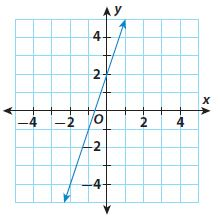__________________

The graph is a straight line but does not pass through the origin. So, the relationship is not proportional.

Complete the table for the equation. Then use the table to graph the equation.

Question 5.
y = x − 1Type below:
____________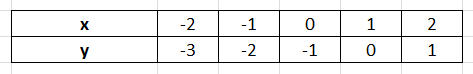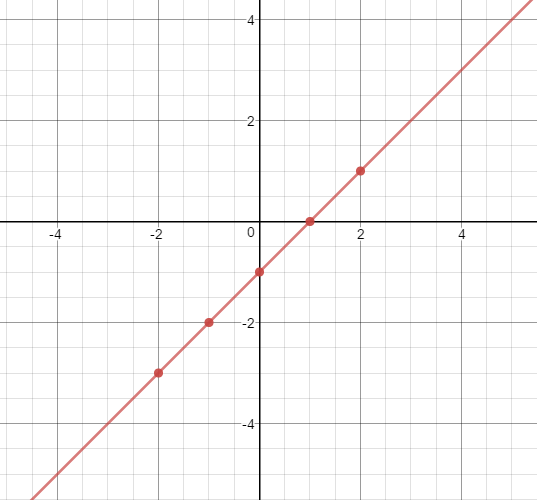Explanation:
y = x – 1
Choose several values of x and substitute in the equation to find y to draw a table.
x = -2; y = -2 – 1 = -2
x = -1; y = -1 -1 = -2
x = 0; y = 0 -1 = -1
x = 1; y = 1 – 1 = 0
x = 2; y = 2 -1 = 1
Also, Plot the ordered pairs from the table. Then draw a line connecting the points to represent all the possible solutions

Essential Question Check-In

Question 6.
How can you choose values for x when making a table of values representing a real-world situation?
Type below:
____________

When choosing values for x in a real-world situation, you choose positive values with an appropriate interval to represent the array of data.

### Independent Practice – Representing Linear Nonproportional Relationships – Page No. 99

State whether the graph of each linear relationship is a solid line or a set of unconnected points. Explain your reasoning.

Question 7.
The relationship between the number of $4 lunches you buy with a$100 school lunch card and the money remaining on the card
____________

Set of unconnected points.

Explanation:
You cannot buy a fractional part of a lunch.
Set of unconnected points.

Question 8.
The relationship between time and the distance remaining on a 3-mile walk for someone walking at a steady rate of 2 miles per hour.
____________

A solid line

Explanation:
The relationship between time and the distance remaining on a 3-mile walk for someone walking at a steady rate of 2 miles per hour. The distance remaining can be a fraction. The time can be in a fraction as well.
A solid line

Nonproportional Relationship Graph Question 9.
Analyze Relationships
Simone paid $12 for an initial year’s subscription to a magazine. The renewal rate is$8 per year. This situation can be represented by the equation y = 8x + 12, where x represents the number of years the subscription is renewed and y represents the total cost.
a. Make a table of values for this situation.Type below:
____________Explanation:
y = 8x + 12
Choose several values for x and substitute in the equation to find y.

Question 9.
b. Draw a graph to represent the situation. Include a title and axis labels.
Type below:
____________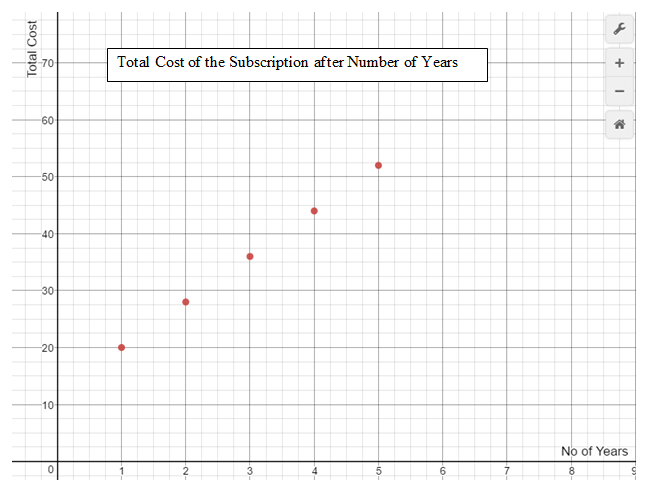Explanation:
Plot the ordered pairs from the table. Then draw a line connecting the points to represent all the possible solutions

Question 9.
c. Explain why this relationship is not proportional.Type below:
____________

It is not proportional as the graph does not pass through the origin

Explanation:
When a relationship is proportional, the graph of the equation passes through the origin.
It is not proportional as the graph does not pass through the origin

Question 9.
d. Does it make sense to connect the points on the graph with a solid line? Explain.
Type below:
____________

No

Explanation:
No; The subscription is renewed for the entire year and cannot be done for a fraction of the year. The number of years must be a whole numb, so the total cost goes up in $8 increments. ### Representing Linear Nonproportional Relationships – Page No. 100 Question 10. Analyze Relationships A proportional relationship is a linear relationship because the rate of change is constant (and equal to the constant of proportionality). What is required of a proportional relationship that is not required of a general linear relationship? Type below: ____________ Answer: The ratio between one quantity to the other quantity should be constant for a proportional linear relationship. The graph should be a straight line that passes through the origin. Lesson 4.1 Representing Linear Nonproportional Relationships Answer Key Question 11. Communicate Mathematical Ideas Explain how you can identify a linear non-proportional relationship from a table, a graph, and an equation. Type below: ____________ Answer: In a table, the ratios y/x will not be equal. A graph will not pass through the origin. An equation will be in the form y = mx + b where b is not equal to 0. Focus on Higher Order Thinking Question 12. Critique Reasoning George observes that for every increase of 1 in the value of x, there is an increase of 60 in the corresponding value of y. He claims that the relationship represented by the table is proportional. Critique George’s reasoning.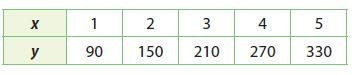Type below: ____________ Answer: The ratio is not constant, hence the relationship cannot be proportional. Explanation: Find y/x 90/1 = 90 150/2 = 75 210/3 = 70 270/4 = 67.5 330/5 = 66 The ratio is not constant, hence the relationship cannot be proportional. Question 13. Make a Conjecture Two parallel lines are graphed on a coordinate plane. How many of the lines could represent proportional relationships? Explain. Type below: ____________ Answer: Maximum one Explanation: When there are two parallel lines, only one can pass through the origin and a line representing a proportional relationship must pass through the origin. Maximum one ### Guided Practice – Determining Slope and y-intercept – Page No. 104 Find the slope and y-intercept of the line in each graph. Question 1.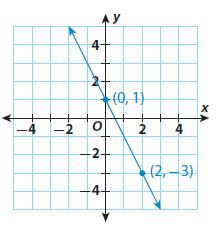slope m = _____ y-intercept b = _____ m = ____________ b = ____________ Answer: slope m = -2 y-intercept b = 1 m = -2 b = 1 Explanation: Find the slope using two points from the grapgh by Slope m = (y2 -y1)/(x2 – x1) where (x1, y1) = (0, 1) and (x2, y2) = (2, -3) Slope m = (y2 -y1)/(x2 – x1) = (-3 – 1)/(2 – 0) = -4/2 = -2 From the graph when x = 0 y-intercept (b) = 1 Lesson 4.2 Determining Slope and Y-Intercept Answer Key Pdf Question 2.slope m = _____ y-intercept b = _____ m = ____________ b = ____________ Answer: slope m = 5 y-intercept b = -15 m = 5 b = -15 Explanation: Find the slope using two points from the graph by Slope m = (y2 -y1)/(x2 – x1) where (x1, y1) = (3, 0) and (x2, y2) = (0, -15) Slope m = (y2 -y1)/(x2 – x1) = (-15 – 0)/(0 – 3) = 15/3 = 5 From the graph when x = 0 y-intercept (b) = -15 Question 3.slope m = _____ y-intercept b = _____ Type below: ____________ Answer: slope m = 3/2 y-intercept b = -2 m = 3/2 b = -2 Explanation: Find the slope using two points from the graph by Slope m = (y2 -y1)/(x2 – x1) where (x1, y1) = (0, -2) and (x2, y2) = (2, 1) Slope m = (y2 -y1)/(x2 – x1) = (1 – (-2))/(2 – 0) = 3/2 From the graph when x = 0 y-intercept (b) = -2 Question 4.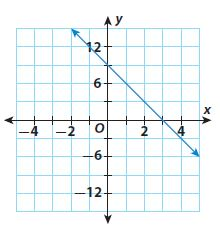slope m = _____ y-intercept b = _____ m = ____________ b = ____________ Answer: slope m = -3 y-intercept b = 9 m = -3 b = 9 Explanation: Find the slope using two points from the grapgh by Slope m = (y2 -y1)/(x2 – x1) where (x1, y1) = (3, 0) and (x2, y2) = (0, 9) Slope m = (y2 -y1)/(x2 – x1) = (9 – 0))/(0 – 3) = -9/3 = -3 From the graph when x = 0 y-intercept (b) = 9 Find the slope and y-intercept of the line represented by each table. Question 5.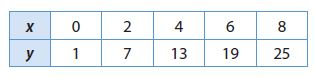slope m = _____ y-intercept b = _____ m = ____________ b = ____________ Answer: slope m = 3 y-intercept b = 1 m = 3 b = 1 Explanation: Find the slope using two points from the grapgh by Slope m = (y2 -y1)/(x2 – x1) where (x1, y1) = (8, 25) and (x2, y2) = (6, 19) Slope m = (y2 -y1)/(x2 – x1) = (19 – 25)/(6 – 8) = 6/2 = 3 From the graph when x = 0 y-intercept (b) = 1 Question 6.slope m = _____ y-intercept b = _____ m = ____________ b = ____________ Answer: slope m = -4 y-intercept b = 140 m = -4 b = 140 Explanation: Find the slope using two points from the grapgh by Slope m = (y2 -y1)/(x2 – x1) where (x1, y1) = (5, 120) and (x2, y2) = (15, 80) Slope m = (y2 -y1)/(x2 – x1) = (80 – 120)/(15 – 5) = -40/10 = -4 From the graph when x = 0 y-intercept (b) = 140 Essential Question Check-In Question 7. How can you determine the slope and the y-intercept of a line from a graph? Type below: ____________ Answer: Choose any two points on the line from the graph and use it to find the slope. Determine the point where the line crosses the y-axis to find the y-intercept. ### Independent Practice – Determining Slope and y-intercept – Page No. 105 Question 8. Some carpet cleaning costs are shown in the table. The relationship is linear. Find and interpret the rate of change and the initial value for this situation.Type below: _____________ Answer: Find the slope using two points Slope m = (y2 -y1)/(x2 – x1) where (x1, y1) = (1, 125) and (x2, y2) = (3, 225) Slope m = (y2 -y1)/(x2 – x1) = (225 – 125)/(3 – 1) = 100/2 = 50 Find the initial value when the value of x is 0 Work backward from x = 1 to x = 0 (175 – 125)/(2 – 1) = 50/1 = 50 Subtract the difference of x and y from the first point. x = 1 – 1 = 0 y = 125 – 50 = 75 y-intercept (b) = 75 The slope/rate of change represents the increase in the cost of cleaning the rooms for a unit increase in the number of rooms. The y-intercept shows the initial cost of carpet cleaning. Question 9. Make Predictions The total cost to pay for parking at a state park for the day and rent a paddleboat are shown.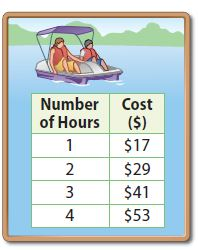a. Find the cost to park for a day and the hourly rate to rent a paddleboat. Type below: _____________ Answer:$5

Explanation:
Find the slope using two points
Slope m = (y2 -y1)/(x2 – x1) where (x1, y1) = (1, 17) and (x2, y2) = (2, 29)
Slope m = (y2 -y1)/(x2 – x1) = (29 – 17)/(2 – 1) = 12/1 = 12
Find the initial value when the value of x is 0
Work backward from x = 1 to x = 0
(29 – 17)/(2 – 1) = 12/1 = 12
Subtract the difference of x and y from the first point.
x = 1 – 1 = 0
y = 17 – 12 = 5
The cost to park for a day is $5. Question 9. b. What will Lin pay if she rents a paddleboat for 3.5 hours and splits the total cost with a friend? Explain.$ _____________

$23.5 Explanation: When Lin paddles for 3.5hr Total Cost = 3.5(12) + 5 = 47 Lin’s cost = 47/2 = 23.5 Question 10. Multi-Step Raymond’s parents will pay for him to take sailboard lessons during the summer. He can take half-hour group lessons or half-hour private lessons. The relationship between cost and number of lessons is linear.a. Find the rate of change and the initial value for the group lessons. Type below: ____________ Answer:$25

Explanation:
Find the slope using two points
Slope m = (y2 -y1)/(x2 – x1) where (x1, y1) = (1, 55) and (x2, y2) = (2, 85)
Slope m = (y2 -y1)/(x2 – x1) = (85 – 55)/(2 – 1) = 30/1 = 30
Rate of change is $30 for per lesson Find the initial value when the value of x is 0 Work backward from x = 1 to x = 0 (85 – 55)/(2 – 1) = 30/1 = 30 Subtract the difference of x and y from the first point. x = 1 – 1 = 0 y = 55 – 30 = 25 The initial value of the group lesson is$25.

Question 10.
b. Find the rate of change and the initial value for the private lessons.
Type below:
_____________

$25 Explanation: Find the slope using two points Slope m = (y2 -y1)/(x2 – x1) where (x1, y1) = (1, 75) and (x2, y2) = (2, 125) Slope m = (y2 -y1)/(x2 – x1) = (125 – 75)/(2 – 1) = 50/1 = 50 The rate of change is$50 per lesson
Find the initial value when the value of x is 0
Work backward from x = 1 to x = 0
(125 – 75)/(2 – 1) = 50/1 = 50
Subtract the difference of x and y from the first point.
x = 1 – 1 = 0
y = 75 – 50 = 25
______ visits

50 visits

Explanation:
You would get 50 visits for $200H.O.T. Focus on Higher Order Thinking Question 14. Explain the Error A student says that the slope of the line for the equation y = 20 − 15x is 20 and the y-intercept is 15. Find and correct the error. Type below: _____________ Answer: The slope is -15 as it represents the change in y per unit change in x. The y-intercept is 20 when x = 0. Explanation: y = 20 − 15x The slope is -15 as it represents the change in y per unit change in x. The y-intercept is 20 when x = 0. Question 15. Critical Thinking Suppose you know the slope of a linear relationship and a point that its graph passes through. Can you graph the line even if the point provided does not represent the y-intercept? Explain. Type below: _____________ Answer: Yes. You can plot the given point and use the slope to find a second point. Connect the points by drawing a line. Question 16. Make a Conjecture Graph the lines y = 3x, y = 3x − 3, and y = 3x + 3. What do you notice about the lines? Make a conjecture based on your observation.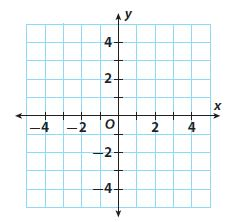Type below: _____________ Answer:Explanation: let’s tale the example y = 3x y = 3x – 3 y = 3x + 3 We notice that the lines are parallel to each other: the slopes of the lines are equal but the y-intersection point differs. ### Guided Practice – Proportional and Nonproportional Situations – Page No. 117 Determine if each relationship is a proportional or nonproportional situation. Explain your reasoning. Question 1.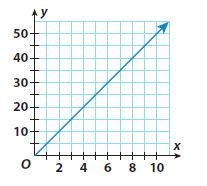Look at the origin. _____________ Answer: Proportional relationship Explanation: Proportional relationship The graph passes through the origin. Graph of a proportional relationship must pass through the origin Question 2.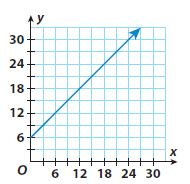_____________ Answer: Non-proportional relationship Explanation: The graph does not pass through the origin. The graph of a proportional relationship must pass through the origin Non-proportional relationship Lesson 4 Skills Practice Proportional and Nonproportional Relationships Answer Key Question 3. q = 2p + $$\frac{1}{2}$$ Compare the equation with y = mx + b. _____________ Answer: q = 2p + $$\frac{1}{2}$$ The equation is in the form y = mx + b, with p being used es the variable instead of x and q instead of y. The value of m is 2, and the value b is 1/2. Since b is not 0, the relationship presented through the above equation is non-proportional. Question 4. v = $$\frac{1}{10}$$u _____________ Answer: Proportional relationship Explanation: v = $$\frac{1}{10}$$u Compare with the form of equation y = mx + b. The equation represents a proportional relationship if b = 0 Proportional relationship ### Proportional and Nonproportional Situations – Page No. 118 The tables represent linear relationships. Determine if each relationship is a proportional or nonproportional situation. Question 5.Find the quotient of y and x. _____________ Answer: proportional relationship Explanation: Find the ratio y/x 12/3 = 4 36/9 = 4 84/21 = 4 Since the ratio is constant, the relationship is proportional. Lesson 4 Skills Practice Proportional and Nonproportional Relationships Question 6.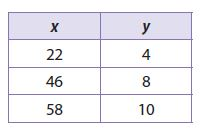_____________ Answer: non-proportional Explanation: Find the ratio y/x 4/22 = 2/11 8/46 = 4/23 10/58 = 5/29 Since the ratio is not constant, the relationship is non-proportional. Question 7. The values in the table represent the number of households that watched three TV shows and the ratings of the shows. The relationship is linear. Describe the relationship in other ways.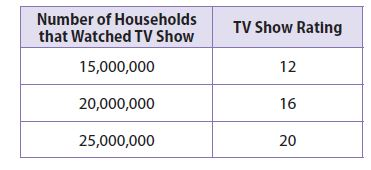Type below: _____________ Answer: proportional relationship Explanation: Find the ratio y/x 12/15,000,000 = 0.0000008 16/20,000,000 = 0.0000008 20/25,000,000 = 0.0000008 Since the ratio is constant, the relationship is proportional. Essential Question Check-In Question 8. How are using graphs, equations, and tables similar when distinguishing between proportional and nonproportional linear relationships? Type below: _____________ Answer: The ratio between y to x is constant when the relationship is proportional. Graphs, tables, and equations all can be used to find the ratio. The ratio is not constant when the relationship is non-proportional. ### Independent Practice – Proportional and Nonproportional Situations – Page No. 119 Question 9. The graph shows the weight of a cross-country team’s beverage cooler based on how much sports drink it contains.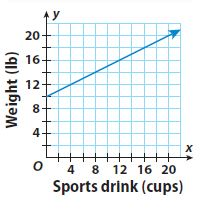a. Is the relationship proportional or nonproportional? Explain. _____________ Answer: Non-proportional Explanation: The graph does not pass through the origin. Graph of a proportional relationship must pass through the origin Non-proportional Question 9. b. Identify and interpret the slope and the y-intercept. Type below: _____________ Answer: Slope m = (y2 -y1)/(x2 – x1) = (12 – 10)/(4 – 0) = 0.5 y-intercept is the weight of the empty cooler, which is 10 lbs. Explanation: Find the slope using two points from the grapgh by Slope m = (y2 -y1)/(x2 – x1) where (x1, y1) = (0, 10) and (x2, y2) = (4, 12) Slope m = (y2 -y1)/(x2 – x1) = (12 – 10)/(4 – 0) = 0.5 From the graph when x = 0 y-intercept (b) = 10 y-intercept is the weight of the empty cooler, which is 10 lbs. In 10–11, tell if the relationship between a rider’s height above the first floor and the time since the rider stepped on the elevator or escalator is proportional or nonproportional. Explain your reasoning. Question 10. The elevator paused for 10 seconds after you stepped on before beginning to rise at a constant rate of 8 feet per second._____________ Answer: Non-proportional Explanation: As there is a pause of 10 seconds, it would be the y-intercept of the graph (when x = 0) Non-proportional Representing Linear Nonproportional Relationships Lesson 4.1 Answer Key Question 11. Your height, h, in feet above the first floor on the escalator is given by h = 0.75t, where t is the time in seconds. _____________ Answer: Proportional Explanation: Comparing with y = mx + b, where b = 0 Proportional Analyze Relationships Compare and contrast the two graphs. Question 12. Graph A Graph B y = $$\frac{1}{3}$$ x y = $$\sqrt { x }$$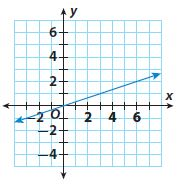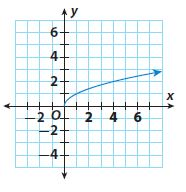Type below: _____________ Answer: Graph A represents a linear relationship while Graph B represents an exponential relationship. They both pass through the origin and the value of y increases with an increase in x. ### Proportional and Nonproportional Situations – Page No. 120 Question 13. Represent Real-World Problems Describe a real-world situation where the relationship is linear and nonproportional. Type below: _____________ Answer: The entrance fee to the amusement park is$8 and there is a fee of \$2 per ride.

H.O.T.

Focus on Higher Order Thinking

Question 14.
Mathematical Reasoning
Suppose you know the slope of a linear relationship and one of the points that its graph passes through. How can you determine if the relationship is proportional or nonproportional?
Type below:
_____________

Use the graph and the given point to determine the second point. Connect the two points by a straight line. If the graph passes through the origin, the relationship is proportional and if the graph does not pass through the origin, the relationship is non-proportional.

Lesson 4 Proportional and Nonproportional Relationships Question 15.
Multiple Representations
An entrant at a science fair has included information about temperature conversion in various forms, as shown. The variables F, C, and K represent temperatures in degrees Fahrenheit, degrees Celsius, and kelvin, respectively.a. Is the relationship between kelvins and degrees Celsius proportional? Justify your answer in two different ways.
_____________

No, the relationship is not proportional.

Explanation:
Compare the equation B to the form: y = mx + b. Since b is not equal to 0, the relationship is non-proportional.
Find the ratio between the Kelvin and Degrees Celsius. Since the ratio is not constant, the relationship is non-proportional.
281.15/8 = 35.14
288.15/15 = 19.21
309.15/36 = 8.59
No, the relationship is not proportional.

Question 15.
b. Is the relationship between degrees Celsius and degrees Fahrenheit proportional? Why or why not?
_____________

No, the relationship is not proportional.

Explanation:
Compare the equation A to the form: y = mx + b. Since b is not equal to 0, the relationship is non-proportional.
No, the relationship is not proportional.

### 4.1 Representing Linear Nonproportional Relationships – Model Quiz – Page No. 121

Lesson 4.1 Representing Linear Nonproportional Relationships Question 1.
Complete the table using the equation y = 3x + 2.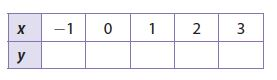Type below:
_____________Explanation:
Given y = 3x + 2x = -1; y = 3(-1) + 2 = -3 + 2 = -1
x = 0; y = 3(0) +2 = 2
x = 1; y = 3(1) + 2 = 3 + 2 = 5
x = 2; y = 3(2) + 2 = 6 + 2 = 8
x = 3: y = 3(3) + 2 = 9 + 2 = 11

4.2 Determining Slope and Y-intercept

Question 2.
Find the slope and y-intercept of the line in the graph.Type below:
_____________

Slope = 3
y-intercept (b) = 1

Explanation:
Find the slope using two points from the grapgh by
Slope m = (y2 -y1)/(x2 – x1) where (x1, y1) = (0, 1) and (x2, y2) = (1, 4)
Slope m = (y2 -y1)/(x2 – x1) = (4 – 1)/(1 – 0) = 3/1
From the graph when x = 0
y-intercept (b) = 1

4.3 Graphing Linear Nonproportional Relationships

Question 3.
Graph the equation y = 2x − 3 using slope and y-intercept.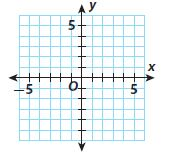Type below:
_____________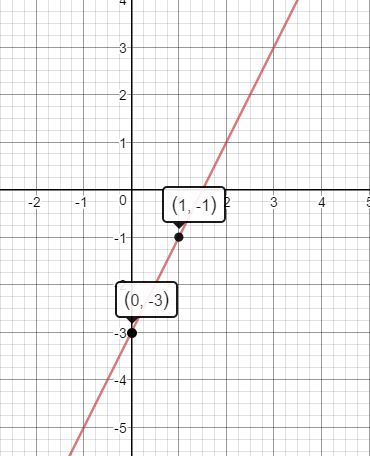Explanation:
Slope = 2
y-intercept = -3
Plot the point that contains the y-intercept (0, -3)
The slope is m = 2/1. Use the slope to find a second point. From (0, -3) count 2 unit up and 1 unit right. The new point is (1, -1)
Draw a line through the points

4.4 Proportional and Nonproportional Situations

Question 4.
Does the table represent a proportional or a nonproportional linear relationship?_____________

Since the ratio is constant, the table represents a proportional linear relationship.

Explanation:
Find the ratio y/x
4/1 = 4
8/2 = 4
12/3 = 4
16/4 = 4
20/5 = 4
Since the ratio is constant, the table represents a proportional linear relationship.

Question 5.
Does the graph in Exercise 2 represent a proportional or a nonproportional linear relationship?
_____________

It represents a non-proportional linear relationship

Explanation:
The line of the graph does not pass through the origin. The graph of a proportional relationship must pass through the origin.
It represents a non-proportional linear relationship

Question 6.
Does the graph in Exercise 3 represent a proportional or a nonproportional relationship?
_____________

It represents a non-proportional linear relationship

Explanation:
The line of the graph does not pass through the origin. The graph of a proportional relationship must pass through the origin
It represents a non-proportional linear relationship

Essential Question

Question 7.
How can you identify a linear nonproportional relationship from a table, a graph, and an equation?
Type below:
_____________

In a table, the ratio of y/x is not constant for a non-proportional relationship.
In a graph, the line of the graph does not pass through the origin for a non-proportional relationship.
In an equation, the b is not equal to y = mx +b for a non-proportional relationship.

### Selected Response – Mixed Review – Page No. 122

Question 1.
The table below represents which equation?Options:
a. y = −x − 10
b. y = −6x
c. y = −4x − 6
d. y = −4x + 2

c. y = −4x − 6

Explanation:
From the table, you can see that the y-intercept (when x = 0) is b = -6. Comparable to y = mx + b
The table is represented by Option C y = -4x – 6

Question 2.
The graph of which equation is shown below?Options:
a. y = −2x + 3
b. y = −2x + 1.5
c. y = 2x + 3
d. y = 2x + 1.5

a. y = −2x + 3

Explanation:
From the table, you can see that the y-intercept (when x = 0) is b = 3. Comparable to y = mx + b
The Option B and D are rejected.
Since the graph is slanting downwards, the slope is negative.
Option C is rejected
The graph represents y = -2x + 3

Question 3.
The table below represents a linear relationship.What is the y-intercept?
Options:
a. -4
b. -2
c. 2
d. 3

b. -2

Explanation:
Find the rate of change
(7 – 4)/(3 – 2) = (10 – 7)/(4 – 3) = 3
Find the value of y for x = 0
Works backward from x = 2 to x = 1
x = 2 – 1 = 1
y = 4 – 3 = 1
x = 1 – 1 = 0
y = 1 – 3 = -2
y-intercept = -2

Question 4.
Which equation represents a nonproportional relationship?
Options:
a. y = 3x + 0
b. y = −3x
c. y = 3x + 5
d. y = $$\frac{1}{3}$$x

c. y = 3x + 5

Explanation:
For a non-proportional relationship, the equation is y = mx + b and b is not equal to 0.
Option C represents a non-proportional relationship y = 3x + 5

Question 5.
The table shows a proportional relationship. What is the missing y-value?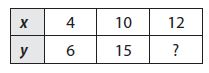Options:
a. 16
b. 20
c. 18
d. 24

c. 18

Explanation:
Find the ratio y/x
6/4 = 3/2
Since the relationship is proportional, the ratio is constant.
Using the ratio to find missing y
3/2 = y/12
y = 3/2 × 12 = 18

Question 6.
What is 0.00000598 written in scientific notation?
Options:
a. 5.98 × 10-6
b. 5.98 × 10-5
c. 59.8 × 10-6
d. 59.8 × 10-7

c. 59.8 × 10-6

Explanation:
0.00000598
Move the decimal 6 points
59.8 × 10-6

Question 7.
The graph shows a linear relationship.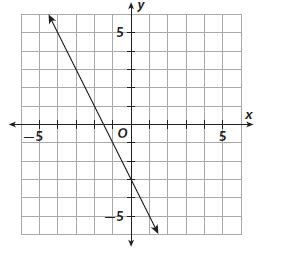a. Is the relationship proportional or nonproportional?
____________

It represents a non-proportional linear relationship

Explanation:
The line of the graph does not pass through the origin. The graph of a proportional relationship must pass through the origin.
It represents a non-proportional linear relationship

Question 7.
b. What is the slope of the line?
_______

Slope m = -2

Explanation:
Find the slope using two points from the graph by
Slope m = (y2 -y1)/(x2 – x1) where (x1, y1) = (0, -2) and (x2, y2) = (2, 1)
Slope m = (y2 -y1)/(x2 – x1) = (-3 -1)/(0 + 2) = -4/2 = -2

Question 7.
c. What is the y-intercept of the line?
_______

y-intercept (b) = -3

Explanation:
From the graph when x = 0
y-intercept (b) = -3

Question 7.
d. What is the equation of the line?
Type below:
____________